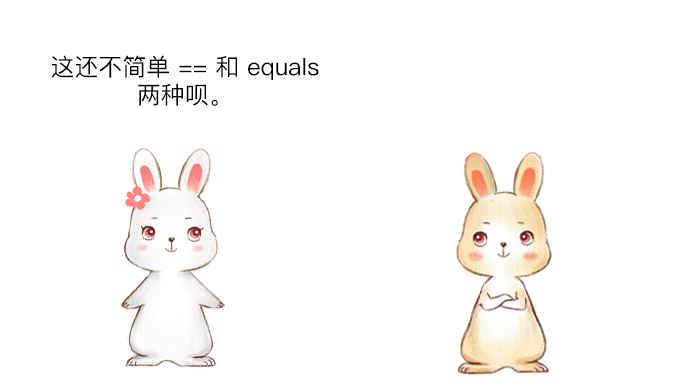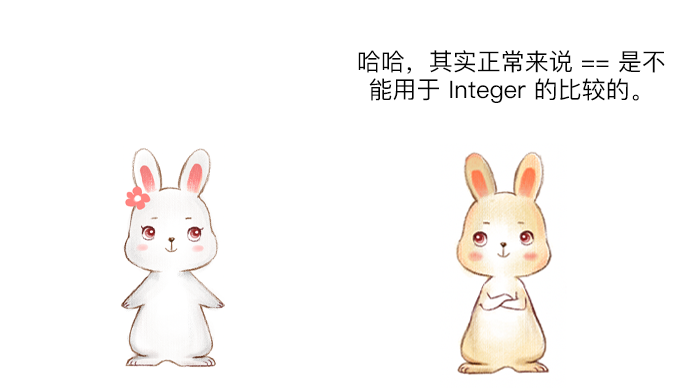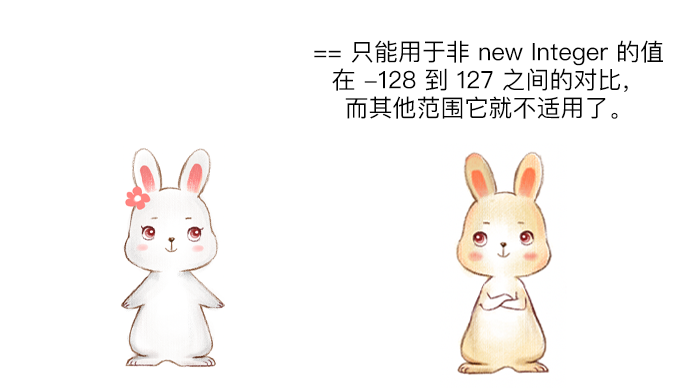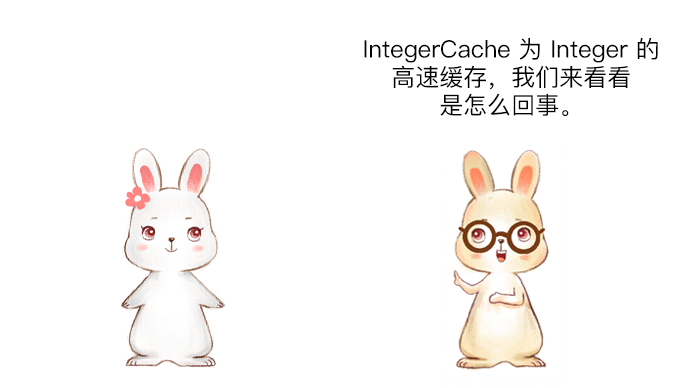# 漫画：Integer 竟然有 4 种比较方法？## 代码测试

public class IntegerTest {
public static void main(String[] args) {
Integer i1 = 127;
Integer i2 = 127;
System.out.println(i1 == i2);
Integer i3 = 128;
Integer i4 = 128;
System.out.println(i3 == i4);
}
}


true

falsepublic class IntegerTest {
public static void main(String[] paramArrayOfString) {
Integer integer1 = Integer.valueOf(127);
Integer integer2 = Integer.valueOf(127);
System.out.println((integer1 == integer2));
Integer integer3 = Integer.valueOf(128);
Integer integer4 = Integer.valueOf(128);
System.out.println((integer3 == integer4));
}
}


public static Integer valueOf(int i) {
if (i >= IntegerCache.low && i <= IntegerCache.high)
return IntegerCache.cache[i + (-IntegerCache.low)];
return new Integer(i);
}


private static class IntegerCache {
static final int low = -128;
static final int high;
static final Integer cache[];

static {
// high value may be configured by property
int h = 127;
String integerCacheHighPropValue =
sun.misc.VM.getSavedProperty("java.lang.Integer.IntegerCache.high");
if (integerCacheHighPropValue != null) {
try {
int i = parseInt(integerCacheHighPropValue);
i = Math.max(i, 127);
// Maximum array size is Integer.MAX_VALUE
h = Math.min(i, Integer.MAX_VALUE - (-low) -1);
} catch( NumberFormatException nfe) {
// If the property cannot be parsed into an int, ignore it.
}
}
high = h;

cache = new Integer[(high - low) + 1];
int j = low;
for(int k = 0; k < cache.length; k++)
cache[k] = new Integer(j++);

// range [-128, 127] must be interned (JLS7 5.1.7)
assert IntegerCache.high >= 127;
}

private IntegerCache() {}
}


【强制】所有整型包装类对象之间值的比较，全部使用 equals 方法比较。

#### 注意事项

public class IntegerTest {
public static void main(String[] args) {
Integer i1 = new Integer(127);
Integer i2 = new Integer(127);
System.out.println(i1 == i2);
}
}


false## 其他比较方式

### compareTo

public final class Integer extends Number implements Comparable<Integer> {
// 忽略其他内容
}


compareTo 的使用如下：

public class IntegerTest {
public static void main(String[] args) {
Integer i1 = new Integer(128);
Integer i2 = new Integer(128);
System.out.println(i1.compareTo(i2));
}
}


0

compareTo 的源码如下：

public int compareTo(Integer anotherInteger) {
return compare(this.value, anotherInteger.value);
}
public static int compare(int x, int y) {
return (x < y) ? -1 : ((x == y) ? 0 : 1);
}


### 直接运算

compareTo 方法给我们了一个启发，我们可以直接将两个值进行相减，如果相减的值等于 0，则说明对比的两个值是相同的，示例代码如下：

public class IntegerTest {
public static void main(String[] args) {
Integer i1 = new Integer(128);
Integer i2 = new Integer(128);
System.out.println((i1 - i2) == 0);
}
}


true

## 扩展知识：IntegerCache 值域修改

IntegerCache 默认的取值范围为 -128 到 127，但我们可以通过设置启动参数来调整 IntegerCache 的最大缓存值，比如我们可以配置虚拟机的启动参数 -XX:AutoBoxCacheMax=1000，此配置表示将缓存的最大值设置为 1000，如果是 Idea 的配置如下：public class IntegerTest {
public static void main(String[] args) {
Integer i1 = 999;
Integer i2 = 999;
System.out.println(i1 == i2);
}
}


true

## 总结## 互动话题

最后的话原创不易，都看到这了，点个「在看」再走呗，这是对我最大的支持与鼓励，谢谢你！PS：公众号推送最近改版了，朋友们设置为星标，防止错过精彩内容。03-0905-19
03-27333
04-082344
10-102420
09-171万+
02-07165
05-046945
02-03162
09-288035